# Electrical Engineering test questions for exams and entrance - 2

## Electrical Engineering test questions for exams and entrance - 2

(1) The width of the diode current pulse in a 2-pulse bridge converter with freewheeling diode is (α is firing angle)

(A) 2α
(B) α/2
(C) π - α
(D) π

(2) Consider a 3-phase induction motor whose rotor power output is 15Kw and corresponding slip is 4%. The rotor copper loss will be

(A) 825W
(B) 725W
(C) 625W
(D) 225W

(3) Four alternators are working in parallel. Rating of each alternator is 5MVA, 11KV with 20% reactance. The short circuit level at bus bars is

(A) 0
(B) 25MVA
(C) 100MVA
(D) 6.25MVA

(4) Which one of the following statement is true? The compensating winding in a dc machine is located

(A) On commutating poles for improving the commutation
(B) On pole shoes to avoid the sparking at the brushes
(C) In armature slots for compensating of the armature reaction
(D) On pole shoes for avoiding the flashover at the commutator surface

ANSWER: On pole shoes for avoiding the flashover at the commutator surface

(5) The number of rotor teeth for a 1.8 step, 4-phase stepper motor that has a total of 40 teeth on 8 poles of stator will be

(A) 100
(B) 50
(C) 80
(D) 40

(6) The magnitude of the voltage developed across the capacitor in a series RLC circuit at resonance

(A) Can be greater than the input voltage, and is in phase with the input voltage
(B) Can be greater than the input voltage, however it is 90° out of phase with the input voltage
(C) Is always zero
(D) Can never be greater than the input voltage

ANSWER: Can be greater than the input voltage, however it is 90° out of phase with the input voltage

(7) ___________is the slowest analog to digital converter

(A) Integrating type
(B) Flash type
(C) Counting type
(D) Successive approximation type

(8) With B open the resistance between Y and G is 6Ω, with Y open the resistance between G and B is 11Ω and with G open the resistance between B and Y is 9Ω. The respective

values of Ry, Rg and Rb are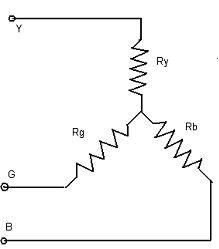(A) 5 Ω, 4 Ω, 6 Ω
(B) 4 Ω, 8 Ω, 7 Ω
(C) 1 Ω, 6 Ω, 5 Ω
(D) 2 Ω, 4 Ω, 7 Ω

ANSWER: 2 Ω, 4 Ω, 7 Ω

(9) MVI X, 10H
MVI Y, 10H
BACK: NOP
RLC
JNC BACK
HLT

The above program is executed in an Intel 8085 processor. The operation NOP is executed __________times.

(A) 1
(B) 3
(C) 5
(D) 2

(10) Consider the figure given below. The source voltage is Vm sin (ωt) and ω=314rad/s. The forward resistance of diode is 5Ω and the remaining parameters are same as that

of an ideal diode. The dc component of source current is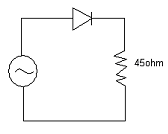(A) 2Vm/100π
(B) 2Vm/50π
(C) Vm/50π
(D) Vm/100π

(11) D flip-flop shown in the figure is triggered at rising edge. The frequency of clock signal is 10KHz. The signal available at Q will have the frequency of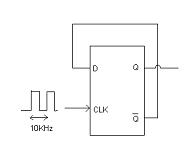(A) 5KHz
(B) 10KHz
(C) 20KHz
(D) 1KHz

(12) Consider an ac machine with 60° phase spread. For this ac machine a 4-pole, 3-phase, double layer winding is housed in a 36-slot stator. Coil span is 7 slot pitches. Number of

slots in which top and bottom layers belong to different phases is

(A) 5
(B) 12
(C) 10
(D) 18

(13) Let L = 10 +3θ - θ²/4 μH be the inductance of a certain moving iron ammeter, where θ` is the defection in radians from the zero position. The control

spring torque is 25 x 10-6 Nm/radian. When the meter carries a current of 5A the deflection of the pointer in radian is

(A) 1.0
(B) 2.0
(C) 1.8
(D) 1.2

(14) To a synchronous generator a hydraulic turbine of 250rpm speed is connected. The number of poles required in a generator, in order to produce power at 50 Hz is

(A) 20
(B) 10
(C) 24
(D) 12

(15) The number of roots in the left half of s plane for the equation, s³ - 4s² + s + 6 = 0 will be

(A) 1
(B) 2
(C) 3
(D) 4

(16) The pressure in the working fluid cycle, in thermal power plants is developed by

(A) Feed water pump
(B) Condenser
(C) Turbine
(D) Super heater

(17) For a power MOSFET, the conduction loss versus device current characteristic is best approximated by

(A) An exponentially decaying function
(B) A rectangular hyperbola
(C) A straight line
(D) A parabola

(18) Consider a three-phase system supplying a balanced load. To measure the total power two wattmeters are connected which reads 10.5Kw and –2.5Kw respectively. The total power and the

power factor respectively are

(A) 8.0Kw, 0.334
(B) 8.0Kw, 0.553
(C) 10Kw, 0.7
(D) 10Kw, 0.45

(19) Consider the figure given below. As seen at the terminal P-Q the Thevenin’s equivalent pair (voltage, impedance) is given by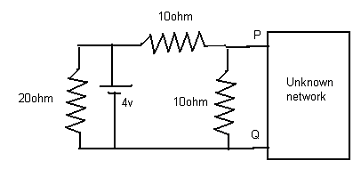(A) 2v, 6ohm
(B) 2v, 5ohm
(C) 4v, 20ohm
(D) 4v, 5ohm

(20) In a Wheatstone bridge R1 and R4 are the opposite arms and R3 and R2 are opposite arms. If a source voltage is applied across R1 and R3, which one of the following is correct under

balanced conditions?

(A) R1 = R2 + R3 + R4
(B) R1 = R3 R4/R2
(C) R1 = R2 R3/R4
(D) R1 = R2 R4/R3

(21) Which one of the following statement is incorrect for the Gauss seidel load flow method?

(A) Initial guess for voltages is essential for convergence
(B) Choice of slack bus affects convergence
(C) Unreliable convergence
(D) Slow convergence

(22) The zero speed regulation at full load without any controller can be provided with

(A) Differential compound dc motor
(B) Cumulative compound dc motor
(C) Series dc motor
(D) Shunt dc motor

For Questions 23 and 24 refer to the data given below: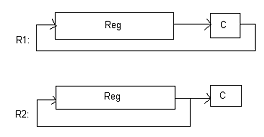R1 and R2 are the two types of rotate right instructions that are available in a microprocessor. Reg is an 8-bit register and C is the carry bit. Rotate left instructions L1 and L2 are

similar except that C now links the most significant bit of Reg instead of the least significant bit.

(23) Let Reg contains 2’s complement number 11010110. What will be the answer if this number is divided by 2?

(A) 00011010
(B) 11101011
(C) 10001000
(D) 11010101

(24) Which set of operation can be used to correctly perform such a division?

(A) L2, R2, R1
(B) R2, L1, R1
(C) R1, L2, R2
(D) L2, R1, R2

(25) What will be the result if distributed winding and short chording are employed in AC machines?

(A) It will increase emf and reduce harmonics
(B) It will reduce emf and increase harmonics
(C) It will reduce both emf and harmonics
(D) It will increase both emf and harmonics